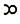### Navigation

Site Navigation

Illustrations

g:geometrical-characters

# Geometrical Characters

+ plus, or more, the sign of addition; signifying that the numbers or quantities between which it is placed are to be added together.

- minus, or less, the sign of subtraction; signifying that the latter of the two quantities between which it is placed is to be taken from the former.

~ denotes the difference of two quantities when it is not known which is the greater.

X into, the sign of multiplication; signifying that the quantities between which it is placed are to be multiplied together.

÷ by, the sign of division; signifying that the former of the two quantities between which it is placed is to be divided by the latter.

: as, or to, :: so is, the sign of an equality of ratios; signifying that the quantities between which they are placed are proportional to each other.

= equal to, the sign of equality; signifying that the quantities between which it is placed are equal to each other.

the radical sign; signifying that the quantity before which it is placed is to have some root of it extracted. — Bonnycastle's Geometry. 12mo. 1823.Formerly used to denote equal to, but is become obsolete.

△ Triangle; as △ ABC = △ ADC.

▭ Rectangled parallelogram.

—: The differences, or excess.

### Page Tools